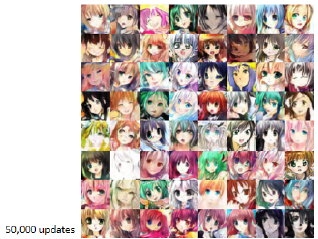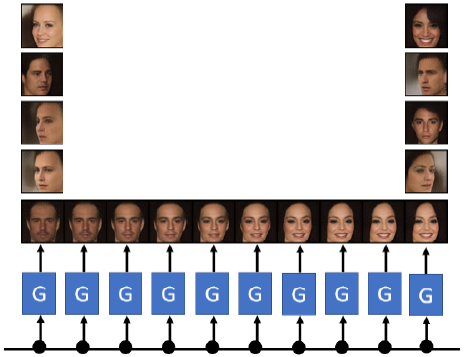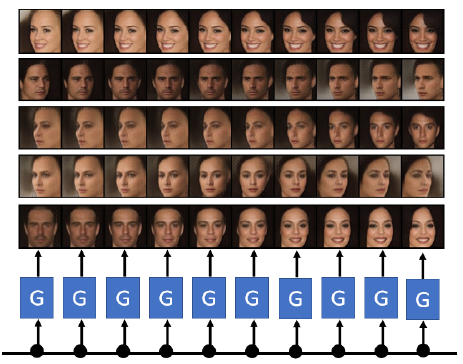# 1 GANs的Generator

### 1.1 Generator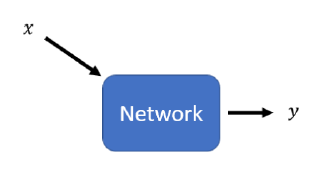$z$通常从正态分布中获取。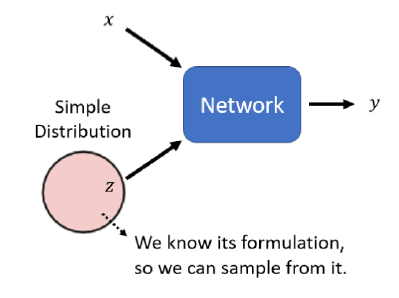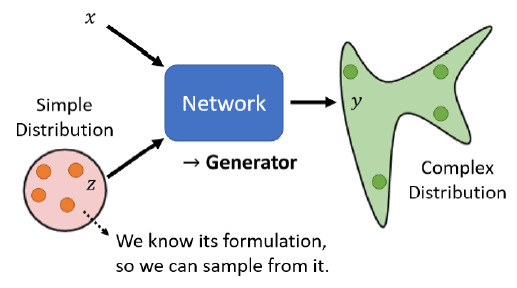### 1.2 Discriminator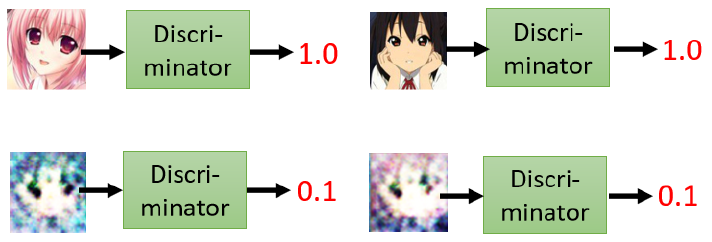discriminator会和generator在训练过程中共同进步。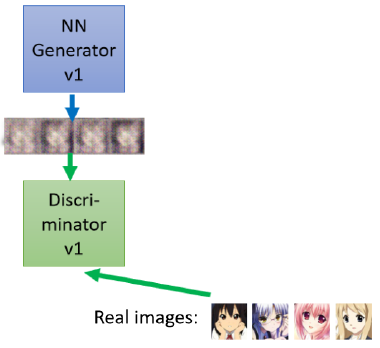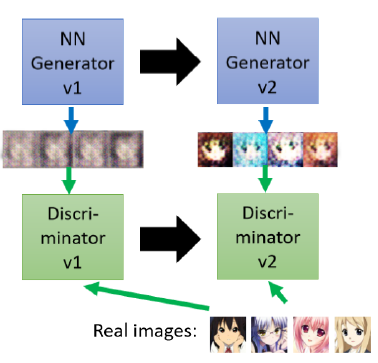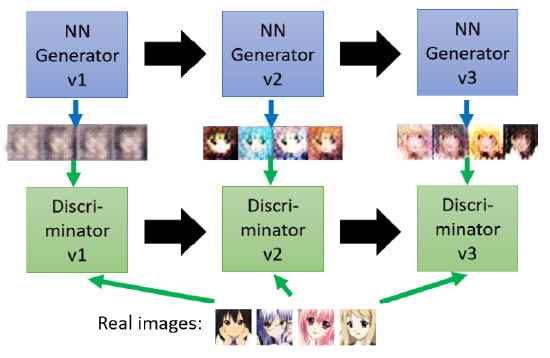# 2 为什么使用随机分布？

• $x$：当前帧$x_i$
• $target$：下一帧$x_{i+1}$
• 算法：supervised learning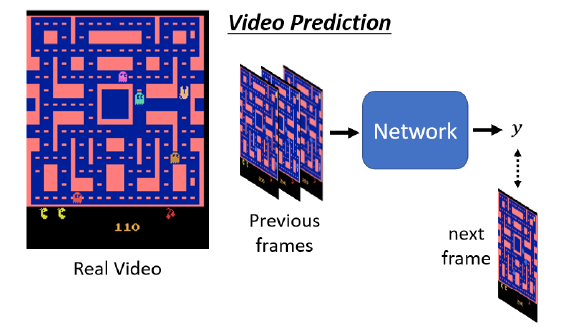• 图像模糊
• 角色可能会突然变换
• 角色转弯时，可能会分裂(因为左右转概率都很高，所以存在两种可能，因此模型两边讨好)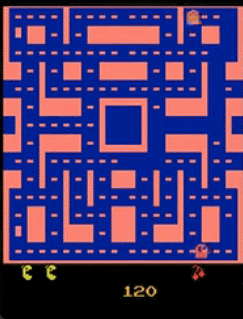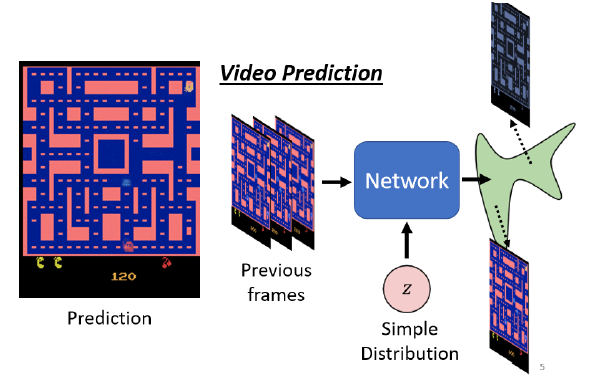# 3 Unconditional/Conditional Generation

Unconditional generation，就是在输入中把$x$拿掉，generator的输入只有$z$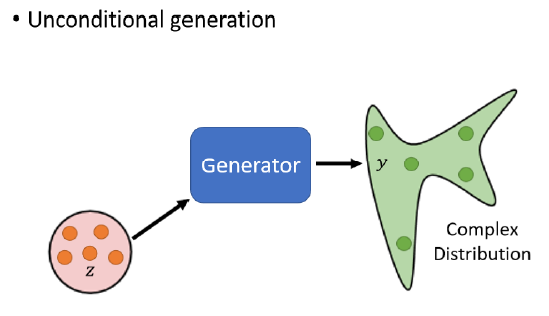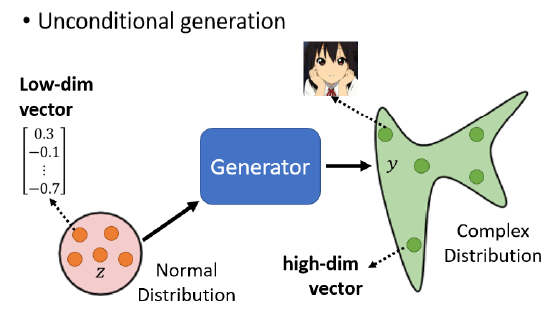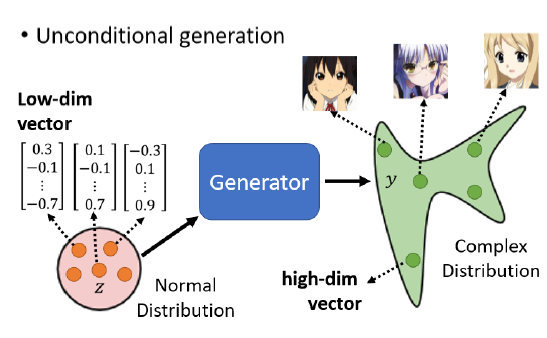# 4 GAN的算法

Generator和discriminator,是两个神经网络，需要相互对抗来进步，因此他们是迭代训练的。

Step 1: 固定 generator G, 然后 update discriminator D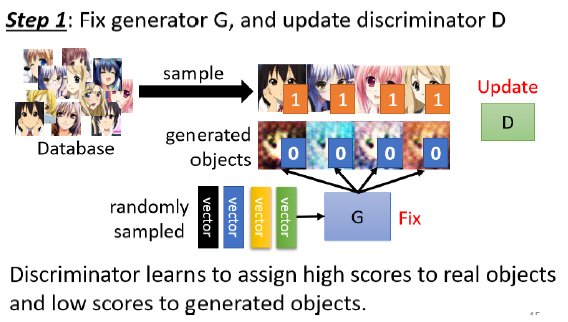Step 2: 固定discriminator D, 然后 update generator G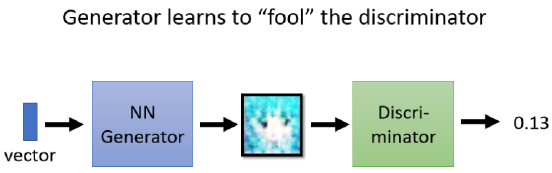• 第一个步骤 固定generator,训练discriminator
• 第二个步骤,固定discriminator训练generator

generator和discriminator会不断变得更强。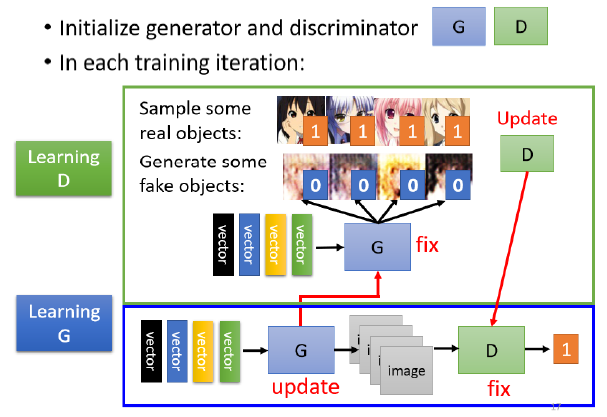# 5 GANs的理论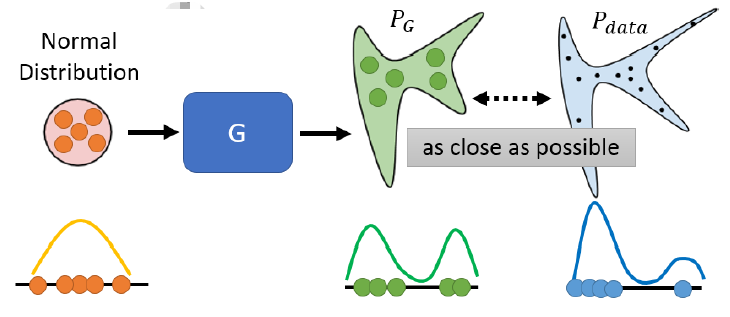• Generator 的 Input 是一个一维的向量
• Generator 的 Output 也是一维的向量
• 真正的 Data 也是一维的向量

Generator的输入是Normal Distribution，分布 如下：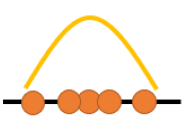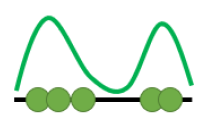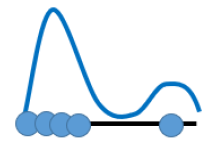$$G^{*}=\arg \min _{G} \operatorname{Div}\left(P_{G}, P_{\text {data }}\right)$$

GAN的解决办法是你不需要知道$P_G$ 跟 $P_{data}$的公式，只要能从 $P_G$ 和 $P_{data}$这两个分布中做sample，就可以计算divergence。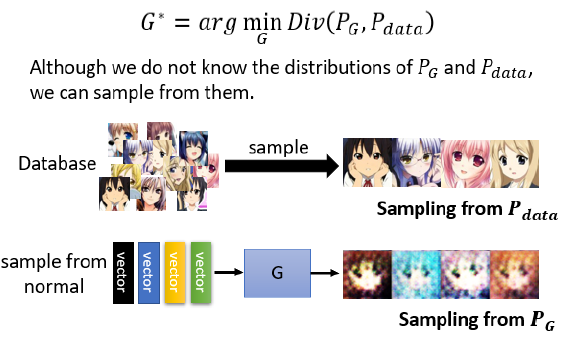• 从真实图片中随机sample一些图片，作为$P_{data}$
• 从normal distribution中做sample，作为generator的输出，而generator的输出就是$P_G$

# 6 Discriminator的优化

Discriminator的目标是最大化Divergence，所以它的objective function是：

$$V(G, D)=E_{y \sim P_{d a t a}}[\log D(y)]+E_{y \sim P_{G}}[\log (1-D(y))]$$

• $E_{y\sim P_{data}}[logD(y)]$ ：输入为真实图片，$D(y)$预测越接近1，则越准确；因此$\log D(y)$越大越好
• $E_{y\sim P_G}[log(1-D(y))]$ 输入为generator生成的图片，$D(y)$预测越接近0越准确；因此，$log(1 - D (y))$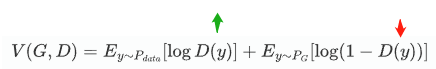\begin{aligned} &G^{*}=\arg \min _{G} \operatorname{Div}\left(P_{G}, P_{d a t a}\right)\\ &D^{*}=\arg \max _{D} V(D, G) \quad \begin{aligned} &\text { The maximum objective value } \\ &\text { is related to JS divergence. } \end{aligned} \end{aligned}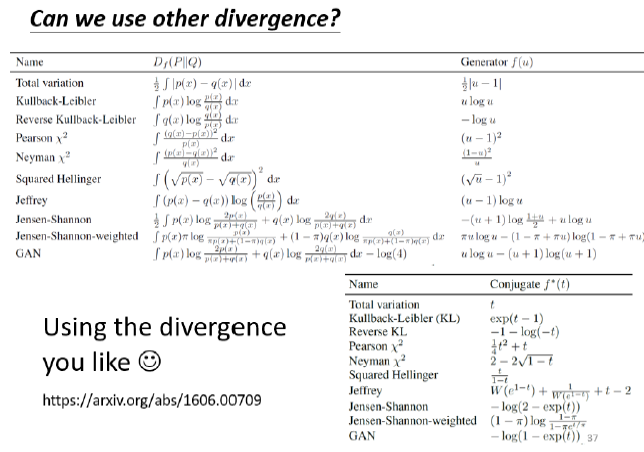# 7 GAN的效果

GAN在某个生成动漫图像任务中的效果如下：

Train 100次：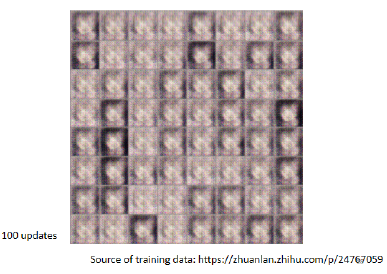Train 1000次：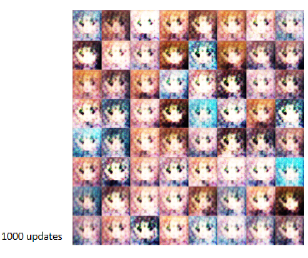Train 2000次：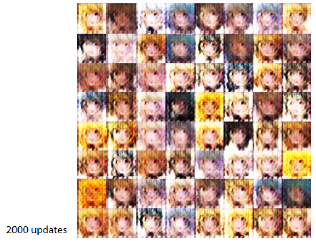Train 5000次：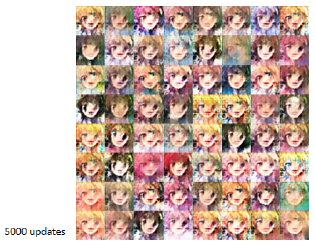Train 10000次：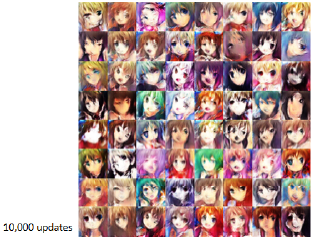Train 20000次：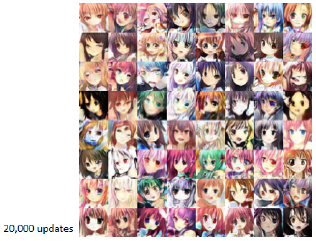Train 50000次：